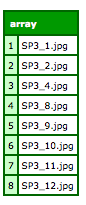Leigh wrote to me with the following problem:

I was wondering if you could give me some advice on how to pick a number out of a file name string and then order the lot by the number in the file name? Eg I have a bunch of images SP1234_1.jpg, SP1234_2.jpg all the way through to SP1234_16.jpg and so on coming out of table.

My issue here is I was ordering by file name out of the DB, works well to 9 images but then ordering goes out of whack at SP1234_10.jpg which then places 2nd.

I know I can grab left() or right() of the file name, but how do i handle the single digit/2 digit numbers?

I assume put those numbers into a list then order by them, but I'm unsure how to pick the number out if it has either 1 or 2 digits?

So what Leigh is seeing here is text based sorting. Text based sorting will sort numbers so that you end up with: 1, 10, 2, etc. Let's show a quick example of that.

`<cfset files = "SP3_9.jpg,SP3_1.jpg,SP3_8.jpg,SP3_10.jpg,SP3_4.jpg,SP3_11.jpg,SP3_12.jpg,SP3_2.jpg"><cfdump var="#listToArray(listSort(files,'text'))#">`

As I didn't have a bunch of files named like Leigh did I simply faked the list with a string. I turned the result into an array to make it a bit nicer to display.If you try to sort these values numerically you get an error. So what to do?

Well Leigh was on the right track about getting the number from the file. But how do you handle the sort then? Once again I'm going to bring up QuickSort, one of my favorite UDFs. QuickSort allows you to define a UDF to compare any two entities. Once you've defined the UDF, you pass it, along with the data, to quickSort, and it handles everything for you.

In our case we need to build a UDF to compare just the numbers from the file names. Here is what I wrote:

`function fileNameCompare(f1, f2) { var num1 = listFirst(listLast(f1, "_"),"."); var num2 = listFirst(listLast(f2, "_"),"."); if(num1 < num2) return -1; if(num1 == num2) return 0; if(num1 > num2) return 1; }`

As you can see, I just use simply list functions to grab the number part. Once I had that the comparison was simple.

So now that I have a UDF, I can sort by converting my list into an array and then passing this all to quickSort:

`<cfset arrFiles = listToArray(files)> <cfdump var="#quickSort(arrFiles,fileNameCompare)#">`

And the result?Here is the complete template I used for testing. One small note. ColdFusion allows you to call UDFs that aren't defined yet in a template. By "yet" I mean on the current line. You can call foo() on line 1 and then define foo() on line 10. You can't do that here though. Since you have to pass the actual UDF instance to quickSort, you have to insure the UDF is defined before you do that. Ok, now the code:

`<cfscript> function fileNameCompare(f1, f2) { var num1 = listFirst(listLast(f1, "_"),"."); var num2 = listFirst(listLast(f2, "_"),"."); if(num1 < num2) return -1; if(num1 == num2) return 0; if(num1 > num2) return 1; }/**Implementation of Hoare's Quicksort algorithm for sorting arrays of arbitrary items.Slight mods by RCamden (added var for comparison)@param arrayToCompare The array to be sorted.@param sorter The comparison UDF.@return Returns a sorted array.@author James Sleeman (james@innovativemedia.co.nz)@version 1, March 12, 2002 */ function quickSort(arrayToCompare, sorter){ var lesserArray = ArrayNew(1); var greaterArray = ArrayNew(1); var pivotArray = ArrayNew(1); var examine = 2; var comparison = 0;pivotArray = arrayToCompare;if (arrayLen(arrayToCompare) LT 2) { return arrayToCompare; }while(examine LTE arrayLen(arrayToCompare)){ comparison = sorter(arrayToCompare[examine], pivotArray); switch(comparison) { case "-1": { arrayAppend(lesserArray, arrayToCompare[examine]); break; } case "0": { arrayAppend(pivotArray, arrayToCompare[examine]); break; } case "1": { arrayAppend(greaterArray, arrayToCompare[examine]); break; } } examine = examine + 1; }if (arrayLen(lesserArray)) { lesserArray = quickSort(lesserArray, sorter); } else { lesserArray = arrayNew(1); }if (arrayLen(greaterArray)) { greaterArray = quickSort(greaterArray, sorter); } else { greaterArray = arrayNew(1); }examine = 1; while(examine LTE arrayLen(pivotArray)){ arrayAppend(lesserArray, pivotArray[examine]); examine = examine + 1; };examine = 1; while(examine LTE arrayLen(greaterArray)){ arrayAppend(lesserArray, greaterArray[examine]); examine = examine + 1; };return lesserArray;} </cfscript><cfset files = "SP3_9.jpg,SP3_1.jpg,SP3_8.jpg,SP3_10.jpg,SP3_4.jpg,SP3_11.jpg,SP3_12.jpg,SP3_2.jpg"><cfdump var="#listToArray(listSort(files,'text'))#"> <p><p> <cfset arrFiles = listToArray(files)> <cfdump var="#quickSort(arrFiles,fileNameCompare)#">`